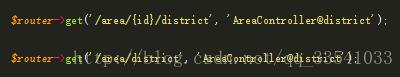更新时间：2019年09月30日 16:24:33   作者：晓风xy残月public function __construct(array \$attributes = [])
{
parent::__construct(\$attributes);

\$this->setParentColumn('pid');
\$this->setOrderColumn('sort');
\$this->setTitleColumn('name');
}

protected function form()
{
return Admin::form(AreaModel::class, function (Form \$form) {

\$form->text('name', '名称')->rules("required");
\$form->select('city_id','城市')->options(

DealCityModel::selectOptionsNoRoot()

\$form->select('pid','地区')->options(array(0 =>'请选择地区'));

});
}

public function district(Request \$request)
{
\$cityId = \$request->get('q');

\$list = AreaModel::where(['city_id' => \$cityId, 'pid' => 0])->get(['id', DB::raw('name as text')]);

foreach (\$list as \$key => \$value) {

\$arr[] = array("id" => \$value->id, "text" => \$value->text);

}

return \$arr;//返回数组到地区的option

}

public function loadOne(\$field, \$sourceUrl, \$idField = 'id', \$textField = 'text')
{
if (Str::contains(\$field, '.')) {
\$field = \$this->formatName(\$field);
\$class = str_replace(['[', ']'], '_', \$field);
} else {
\$class = \$field;
}

\$script = <<<EOT
\$(function(){
var target = \$(".\$class");
\$.get("\$sourceUrl?q="+\$("{\$this->getElementClassSelector()}").val(), function (data) {
target.find("option").remove();
\$(target).select2({
data: \$.map(data, function (d) {
d.id = d.\$idField;
d.text = d.\$textField;
return d;
})
});
});
});
EOT;

return \$this;
}